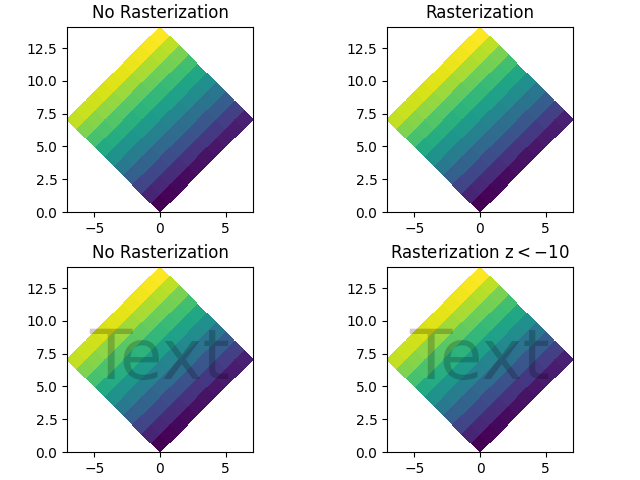# Rasterization for vector graphics¶

Rasterization converts vector graphics into a raster image (pixels). It can speed up rendering and produce smaller files for large data sets, but comes at the cost of a fixed resolution.

Whether rasterization should be used can be specified per artist. This can be useful to reduce the file size of large artists, while maintaining the advantages of vector graphics for other artists such as the axes and text. For instance a complicated pcolormesh or contourf can be made significantly simpler by rasterizing. Setting rasterization only affects vector backends such as PDF, SVG, or PS.

Rasterization is disabled by default. There are two ways to enable it, which can also be combined:

The storage size and the resolution of the rasterized artist is determined by its physical size and the value of the dpi parameter passed to savefig.

Note

The image of this example shown in the HTML documentation is not a vector graphic. Therefore, it cannot illustrate the rasterization effect. Please run this example locally and check the generated graphics files.

import numpy as np
import matplotlib.pyplot as plt

d = np.arange(100).reshape(10, 10)  # the values to be color-mapped
x, y = np.meshgrid(np.arange(11), np.arange(11))

theta = 0.25*np.pi
xx = x*np.cos(theta) - y*np.sin(theta)  # rotate x by -theta
yy = x*np.sin(theta) + y*np.cos(theta)  # rotate y by -theta

fig, ((ax1, ax2), (ax3, ax4)) = plt.subplots(2, 2, constrained_layout=True)

# pcolormesh without rasterization
ax1.set_aspect(1)
ax1.pcolormesh(xx, yy, d)
ax1.set_title("No Rasterization")

# pcolormesh with rasterization; enabled by keyword argument
ax2.set_aspect(1)
ax2.set_title("Rasterization")
m = ax2.pcolormesh(xx, yy, d, rasterized=True)

# pcolormesh with an overlaid text without rasterization
ax3.set_aspect(1)
ax3.pcolormesh(xx, yy, d)
ax3.text(0.5, 0.5, "Text", alpha=0.2,
va="center", ha="center", size=50, transform=ax3.transAxes)
ax3.set_title("No Rasterization")

# pcolormesh with an overlaid text without rasterization; enabled by zorder.
# Setting the rasterization zorder threshold to 0 and a negative zorder on the
# pcolormesh rasterizes it. All artists have a non-negative zorder by default,
# so they (e.g. the text here) are not affected.
ax4.set_aspect(1)
m = ax4.pcolormesh(xx, yy, d, zorder=-10)
ax4.text(0.5, 0.5, "Text", alpha=0.2,
va="center", ha="center", size=50, transform=ax4.transAxes)
ax4.set_rasterization_zorder(0)
ax4.set_title("Rasterization z$<-10$")

# Save files in pdf and eps format
plt.savefig("test_rasterization.pdf", dpi=150)
plt.savefig("test_rasterization.eps", dpi=150)

if not plt.rcParams["text.usetex"]:
plt.savefig("test_rasterization.svg", dpi=150)
# svg backend currently ignores the dpiOut:

The PostScript backend does not support transparency; partially transparent artists will be rendered opaque.


References

The use of the following functions, methods, classes and modules is shown in this example:

Total running time of the script: ( 0 minutes 1.674 seconds)

Keywords: matplotlib code example, codex, python plot, pyplot Gallery generated by Sphinx-Gallery